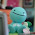## Wednesday, January 26, 2011

### Jayvee's Surface Area Scribe Post

2. A right rectangular prism has six faces. Why might you have to find the area of only three of the faces to be able to find the surface area? Use pictures and words to explain your thinking.

You only need to find the surface area of 3 faces because the top and bottom are the same, as well as the front and back, and the 2 sides.
.4. Find the surface area of this CD case.
Front: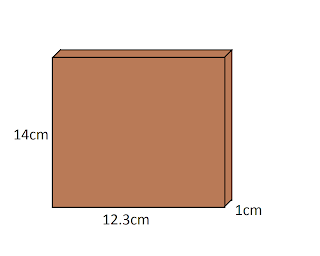14x12.3 = 172.2cm2
14x12.3 = 172.2cm2

Top :
12.3x1 = 12.3cm2
12.3x1 = 12.3cm2

Side :
14x1 = 14cm2
14x1 = 14cm2

TSA = 397cm2

6. Cheese is sometimes packaged in a triangular box. How much cardboard would you need to cover this piece of cheese if you do not include overlapping? Calculate your answer to the nearest tenth of a square centimetre.Front :
9.1x6.4 / 2 = 29.12cm2
9.1x6.4 / 2 = 29.12cm2

Top(Bottom) :
9.1x3 = 27.3cm2

Sides :
6.4x3 = 19.2cm2
6.4x3 = 19.2cm2

TSA = 123.94cm2

8. Paco builds a glass greenhouse.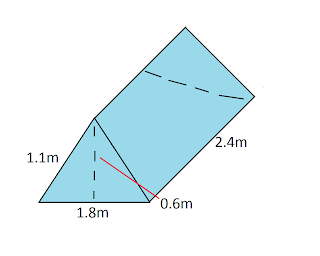A) How many glass faces does the greenhouse have?
The greenhouse have 5 faces.

B) How much glass does Paco need to buy?

Front :
1.8x1.1 / 2 = 0.99m2
1.8x1.1 / 2 = 0.99m2

Top (Bottom) :
2.4x1.8 = 4.32m2

Side :
2.4x1.1 = 2.64m2
2.4x1.1 = 2.64m2

TSA = 11.58m2

My Volume Question :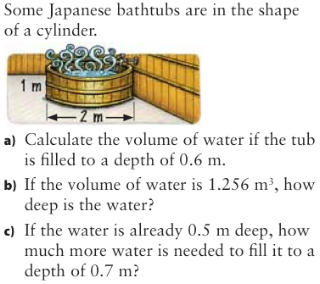Question from 7.3
A) d/2 = r
2/2 = r
1 = r
v = PiexRxRxH
v = 3.14x1x1x0.6
v = 3.14x0.6
v = 1.884cm^3
B) 1.256/1(h) = 1.256
1.256 / 1(r) / 1(r) / 3.14 = 0.4m
C) First, find the volume if the water's depth is 0.7m
3.14 x 1(r) x 1(r) x 0.7 = 2.198m^2
Now, find the volume if the water's depth is 0.5m
3.14 x 1(r) x 1(r) x 0.7 = 1.5 = 1.57m^2
After you get both of the answers, subtract 1.57 from 2.198 = 0.62m^m3

Question from 7.4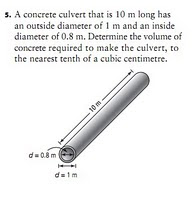First, find the volume of the bigger circle.
3.14 x 0.5 x 0.5 x 10 = 7.85m^2
Now, find the volume of the smaller circle.
3.14 x 0.4 x 0.4 x 10 = 5.024m^2
After you got both volumes, subtract 5.024 from 7.85
7.85 - 5.024 = 2.826m^3

1.2.